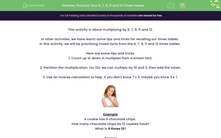# Practise Your 6, 7, 9, 11 and 12 Times tables

In this worksheet, students will learn to recall 6, 7, 9, 11 and 12 times tables.Key stage:  KS 2

Curriculum topic:   Number: Multiplication and Division

Curriculum subtopic:   Know Multiplication Tables up to 12 x 12

Difficulty level:#### Worksheet Overview

This activity is about multiplying by 6, 7, 9, 11 and 12.

In other activities, we have learnt some tips and tricks for recalling our times tables.

In this activity, we will be practising mixed facts from the 6, 7, 9, 11 and 12 times tables.

Here are some tips and tricks:

1. Count up or down in multiples from a known fact.

2. Partition the multiplication. For 12s, we can multiply by 10 and 2, then add the totals.

3. Use an inverse calculation to help. If you don't know 7 x 3, maybe you know 3 x 7.Example

A cookie has 9 chocolate chips.

How many chocolate chips do 12 cookies have?

What is 9 times 12?

Step 1: 9 x 10 = 90

Step 2: 9 x 2 = 18

Step 3: 90 +18 = 108

9 × 12 = 108

Now try the questions for yourself.### What is EdPlace?

We're your National Curriculum aligned online education content provider helping each child succeed in English, maths and science from year 1 to GCSE. With an EdPlace account you’ll be able to track and measure progress, helping each child achieve their best. We build confidence and attainment by personalising each child’s learning at a level that suits them.

Get started••••